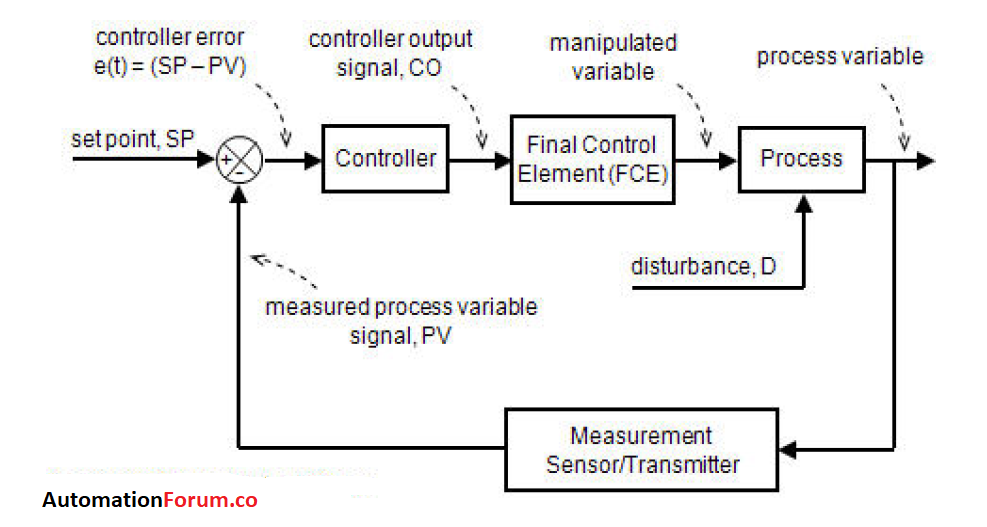Control System

# Continuous control mode- P, PD, PI & PID control modesThe control actions most commonly used in process control are one or a combination of the continuous process controller mode. In these modes, the output of the controller changes smoothly in response to an error or error change rate. There are three basic controller modes, the proportional controller (P), the integral controller (I), the derived controller (D). There are three other combinations of basic controller modes, PI, PD, PID. The PID controller is the most flexible controller.

### P (Proportional) control mode:

The simplest algorithm in the PID family is a proportional or P-Only controller. Like all automatic controllers, it repeats a measurement-computation-action procedure at every loop sample time, T.This is a simple control system when the process variable changes the parameter is feedbacked to the controller. At controller, the PV is compared with the setpoint and takes the appropriate control action. This is proportional control action.

### P-I (proportional and integrative) control mode:

A P-I controller accumulates the error as time passes and is multiplied by the constant Ki. That is your term. It outputs the sum of your P and I terms. PI controllers have two adjustment parameters to adjust. The integral action allows the PI controllers to eliminate the offset, a major weakness of a P-only controller. Therefore, PI controllers provide a balance of complexity and capacity that makes them the most used algorithm in process control applications. This driver is used mainly in areas where system speed is not a problem. Since the P-I controller does not have the ability to predict future system errors, it can not decrease the rise time and eliminate oscillations.

### P-D (proportional-derivative) controller mode:

The objective of using the P-D controller is to increase the stability of the system by improving the control since it has the ability to predict the future error of the system response. This system can not eliminate the displacement of the proportional controllers, however, it can handle rapid process load changes as long as the load change compensation error is acceptable.

The D mode is designed to be proportional to the change of the output variable to avoid the sudden changes that occur in the control output as a result of sudden changes in the error signal. In addition, D directly amplifies the process noise, so the D-only control is not used.

### P-I-D Controller:

The P-I-D controller has the optimal control dynamics that include zero steady-state error, fast response (short rise time), no oscillations and greater stability. With the addition of a third parameter adjustable adjustment derivative, the number of algorithm permutations increases markedly. And there are even different forms of the PID equation itself. This creates additional challenges for the design and adjustment of the driver. One of the main advantages of the P-I-D controller is that it can be used with higher order processes that include more than individual energy storage.

The time response of the controlled variable can be modified by adjusting the proportional gain K, and the integrating and derivative time constants Ti and Td, respectively; the objective is to achieve a zero steady state control error e (t) regardless of whether it is caused by changes in the reference  or the perturbation d(t).

The analytic expression includes the expression of the three parameters: